# Class 8 NCERT Solutions – Chapter 4 Practical Geometry – Exercise 4.5

• Last Updated : 20 Nov, 2020

### 1. The square READ with RE = 5.1 cm.

Steps of construction:

Step 1: Draw one side of the square RE = 5.1 cm.

Attention reader! All those who say programming isn't for kids, just haven't met the right mentors yet. Join the  Demo Class for First Step to Coding Coursespecifically designed for students of class 8 to 12.

The students will get to learn more about the world of programming in these free classes which will definitely help them in making a wise career choice in the future.

Step 2: From E draw an angle of 90°.

Step 3: From E cut EA of 5.1 cm.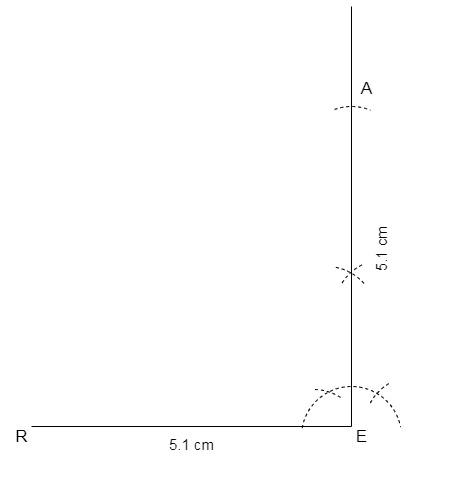Step 4: Now draw an arc of 5.1 cm from both A and R, intersect them and mark the intersection point as D.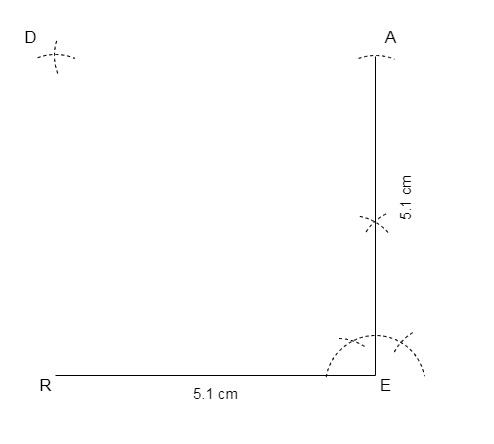Step 5: Join RD and ED.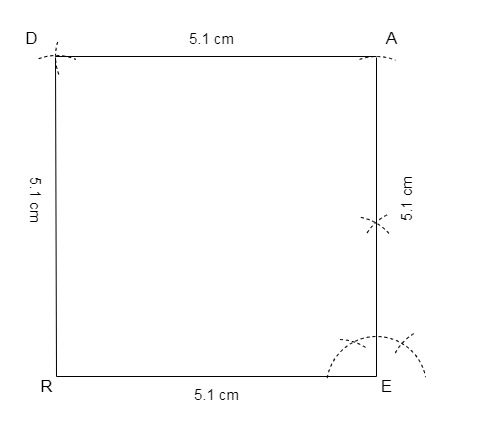Thus, we have the required square READ.

### 2. A rhombus whose diagonals are 5.2 cm and 6.4 cm long.

Steps of construction:

Let the rhombus be ABCD with diagonals, AC = 5.2 cm and BD = 6.4 cm.

Step 1: Draw a line AC of 5.2 cm

Step 2: Draw perpendicular bisector of AC and mark the bisector point as O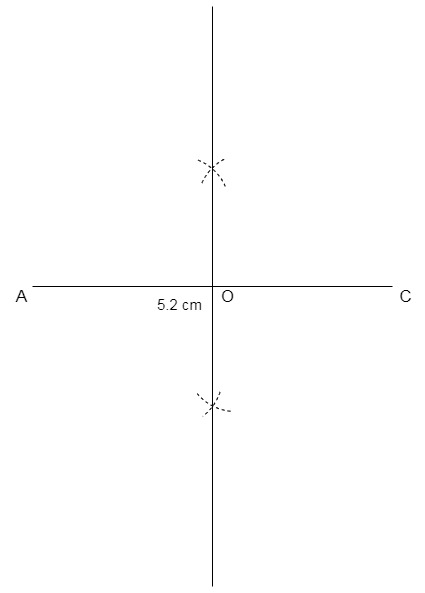Step 3: Draw two arcs with centre O of radius 1/2 × BD = 1/2 × 6.4 cm = 3.2 cm and these arcs will meet the bisector at point B and D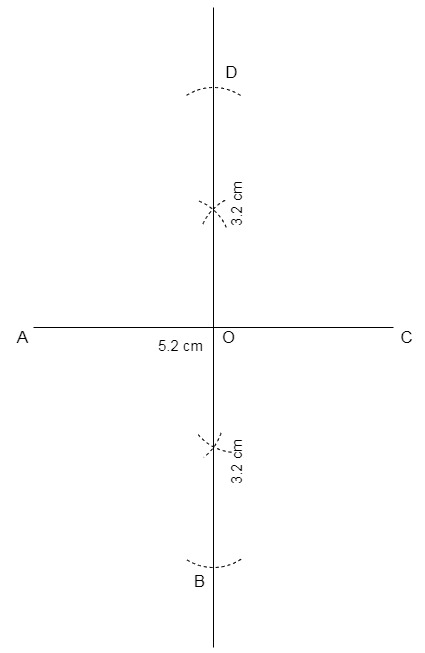Step 4: Join AB, BC, CD and AD.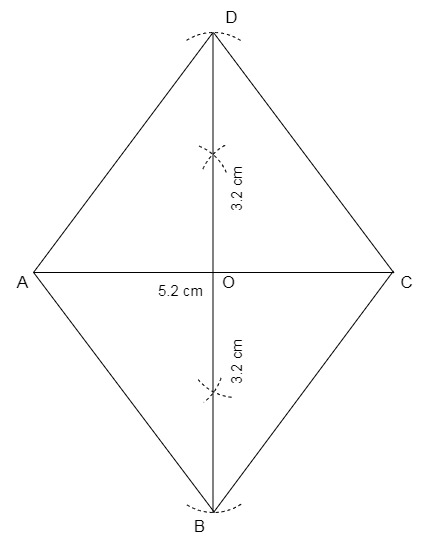Hence, we have the required rhombus ABCD.

### 3. A rectangle with adjacent sides of lengths 5 cm and 4 cm.

Steps of construction:

Step 1: Let the rectangle be CODE with adjacent sides CO = 5 cm and OD = 4 cm.

Step 2: Draw CO = 5 cm.

Step 3: From O draw an angle of 90°.

Step 4: From O cut OD = 4 cm.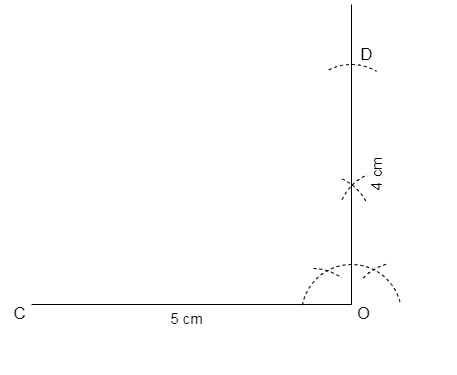Step 5: From C draw an arc of 4 cm and from D draw an arc of 5 cm, intersect both the arcs and mark the point as E.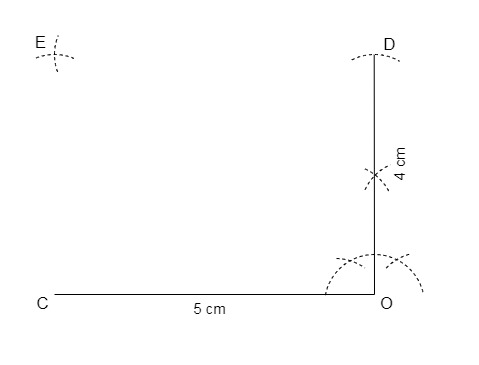Step 6: Join CE and DE.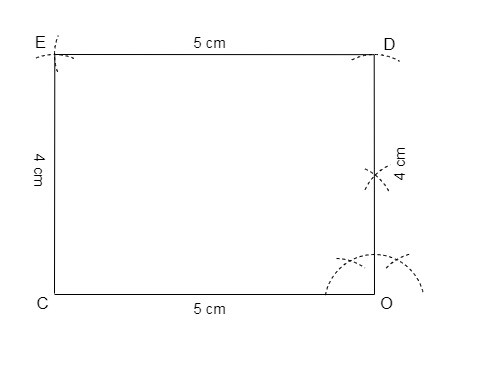Thus, we have the required rectangle CODE.

### 4. A parallelogram OKAY where OK = 5.5 cm and KA = 4.2 cm. Is it unique?

Steps of construction:

Step 1: Draw a line OK of 5.5 cm.

Step 2: Draw a ray at K at any convenient angle, let the angle be 60°.

Step 3: Now cut the ray at 4.2 cm and mark the point as A.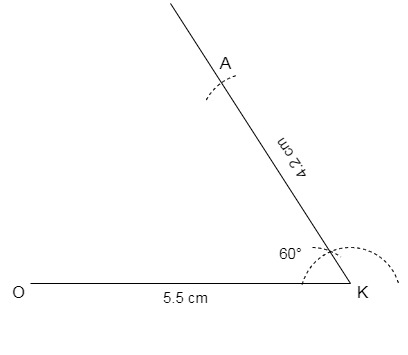Step 4: From A draw an arc of 5.5 cm and from O draw an arc of 4.2 cm, intersect both the arcs at Y.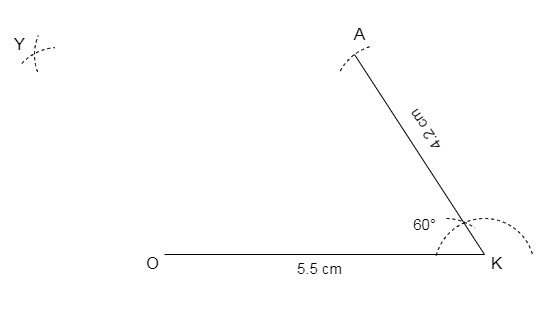Step 5: Join OY and AY.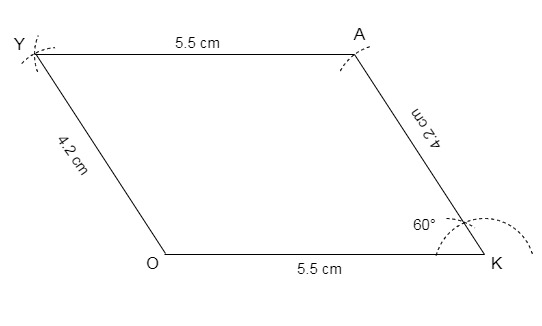Thus, we have the required parallelogram OKAY.
No, it is not unique as angle K can be any angle.

My Personal Notes arrow_drop_up